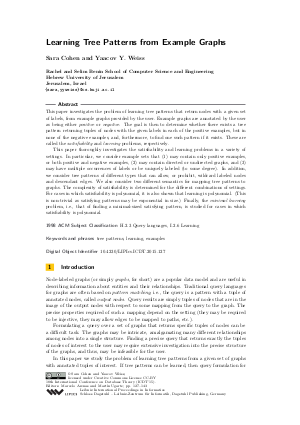Document# Learning Tree Patterns from Example Graphs

### Authors Sara Cohen, Yaacov Y. Weiss## File

LIPIcs.ICDT.2015.127.pdf
• Filesize: 456 kB
• 17 pages

## Cite As

Sara Cohen and Yaacov Y. Weiss. Learning Tree Patterns from Example Graphs. In 18th International Conference on Database Theory (ICDT 2015). Leibniz International Proceedings in Informatics (LIPIcs), Volume 31, pp. 127-143, Schloss Dagstuhl - Leibniz-Zentrum für Informatik (2015)
https://doi.org/10.4230/LIPIcs.ICDT.2015.127

## Abstract

This paper investigates the problem of learning tree patterns that return nodes with a given set of labels, from example graphs provided by the user. Example graphs are annotated by the user as being either positive or negative. The goal is then to determine whether there exists a tree pattern returning tuples of nodes with the given labels in each of the positive examples, but in none of the negative examples, and, furthermore, to find one such pattern if it exists. These are called the satisfiability and learning problems, respectively. This paper thoroughly investigates the satisfiability and learning problems in a variety of settings. In particular, we consider example sets that (1) may contain only positive examples, or both positive and negative examples, (2) may contain directed or undirected graphs, and (3) may have multiple occurrences of labels or be uniquely labeled (to some degree). In addition, we consider tree patterns of different types that can allow, or prohibit, wildcard labeled nodes and descendant edges. We also consider two different semantics for mapping tree patterns to graphs. The complexity of satisfiability is determined for the different combinations of settings. For cases in which satisfiability is polynomial, it is also shown that learning is polynomial (This is non-trivial as satisfying patterns may be exponential in size). Finally, the minimal learning problem, i.e., that of finding a minimal-sized satisfying pattern, is studied for cases in which satisfiability is polynomial.
##### Keywords
• tree patterns
• learning
• examples

## Metrics

• Access Statistics
• Total Accesses (updated on a weekly basis)
0